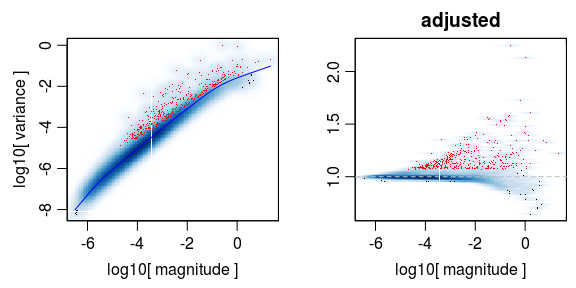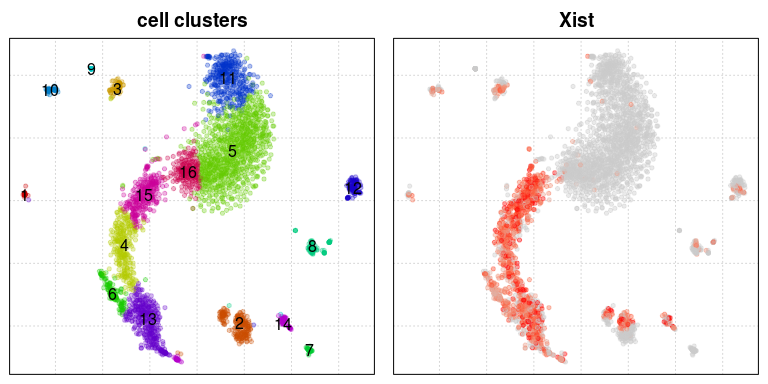Here we show an example of how loom-annotated matrices of a 10x dataset can be loaded and analyzed in R using velocyto.R and pagoda2.

``library(velocyto.R)``
``Loading required package: Matrix``

``````# you can download 10X43_1.loom from the following URL: http://pklab.med.harvard.edu/velocyto/DG1/10X43_1.loom

## Normalize and cluster cells using pagoda2

Using spliced expression matrix as input to pagoda2.

``````emat <- ldat\$spliced
# this dataset has already been pre-filtered, but this is where one woudl do some filtering
emat <- emat[,colSums(emat)>=1e3]``````

## Pagoda2 processing

Pagoda2 is used to generate cell embedding, cell clustering, as well as a more accurate cell-cell distance matrix. You can alternatively generate those using other tools, such as Seurat2, etc.

``library(pagoda2)``
``````

Attaching package: ‘pagoda2’

The following object is masked from ‘package:velocyto.R’:

armaCor``````
``r <- Pagoda2\$new(emat,modelType='plain',trim=10,log.scale=T)``
``3396 cells, 10995 genes; normalizing ... using plain model winsorizing ... log scale ... done.``
``r\$adjustVariance(plot=T,do.par=T,gam.k=10)``
``calculating variance fit ... using gam 388 overdispersed genes ... 388 persisting ... done.``Run basic analysis steps to generate cell embedding and clustering, visualize:

``r\$calculatePcaReduction(nPcs=100,n.odgenes=3e3,maxit=300)``
``running PCA using 3000 OD genes ..``
``Loading required package: irlba``
``.. done``
``r\$makeKnnGraph(k=30,type='PCA',center=T,distance='cosine');``
``````Loading required package: igraph

Attaching package: ‘igraph’

The following objects are masked from ‘package:stats’:

decompose, spectrum

The following object is masked from ‘package:base’:

union``````
``````creating space of type angular done
building index ... done
querying ... done``````
``````r\$getKnnClusters(method=multilevel.community,type='PCA',name='multilevel')
r\$getEmbedding(type='PCA',embeddingType='tSNE',perplexity=50,verbose=T)``````
``````calculating distance ... pearson ...running tSNE using 16 cores:
Read the 3396 x 3396 data matrix successfully!
OpenMP is working...
Using no_dims = 2, perplexity = 50.000000, and theta = 0.500000
Computing input similarities...
Building tree...
Done in 16.31 seconds (sparsity = 0.064151)!
Learning embedding...
Iteration 50: error is 76.140074 (50 iterations in 3.51 seconds)
Iteration 100: error is 65.211975 (50 iterations in 3.15 seconds)
Iteration 150: error is 64.292740 (50 iterations in 3.26 seconds)
Iteration 200: error is 64.022286 (50 iterations in 3.25 seconds)
Iteration 250: error is 63.919209 (50 iterations in 3.53 seconds)
Iteration 300: error is 1.723760 (50 iterations in 3.32 seconds)
Iteration 350: error is 1.520047 (50 iterations in 3.26 seconds)
Iteration 400: error is 1.437406 (50 iterations in 3.37 seconds)
Iteration 450: error is 1.395372 (50 iterations in 3.31 seconds)
Iteration 500: error is 1.376935 (50 iterations in 3.34 seconds)
Iteration 550: error is 1.366568 (50 iterations in 3.31 seconds)
Iteration 600: error is 1.359509 (50 iterations in 3.53 seconds)
Iteration 650: error is 1.354987 (50 iterations in 3.37 seconds)
Iteration 700: error is 1.351789 (50 iterations in 2.89 seconds)
Iteration 750: error is 1.349355 (50 iterations in 3.54 seconds)
Iteration 800: error is 1.346523 (50 iterations in 3.46 seconds)
Iteration 850: error is 1.343971 (50 iterations in 3.52 seconds)
Iteration 900: error is 1.342161 (50 iterations in 2.87 seconds)
Iteration 950: error is 1.340376 (50 iterations in 3.61 seconds)
Iteration 1000: error is 1.338698 (50 iterations in 3.49 seconds)
Fitting performed in 66.87 seconds.``````

Plot embedding, labeling clusters (left) and “Xist” expression (which separates the male and female )

``````par(mfrow=c(1,2))
r\$plotEmbedding(type='PCA',embeddingType='tSNE',show.legend=F,mark.clusters=T,min.group.size=10,shuffle.colors=F,mark.cluster.cex=1,alpha=0.3,main='cell clusters')
r\$plotEmbedding(type='PCA',embeddingType='tSNE',colors=r\$counts[,"Xist"],main='Xist')  ``````
``treating colors as a gradient with zlim: 0 1.192859 ``## Velocity estimation

Prepare matrices and clustering data:

``````emat <- ldat\$spliced; nmat <- ldat\$unspliced
emat <- emat[,rownames(r\$counts)]; nmat <- nmat[,rownames(r\$counts)]; # restrict to cells that passed p2 filter
# take cluster labels
cluster.label <- r\$clusters\$PCA[]
cell.colors <- sccore::fac2col(cluster.label)
# take embedding
emb <- r\$embeddings\$PCA\$tSNE``````

In addition to clustering and the t-SNE embedding, from the p2 processing we will also take a cell-cell distance, which will be better than the default whole-transcriptome correlation distance that velocyto.R would normally use.

``cell.dist <- as.dist(1-armaCor(t(r\$reductions\$PCA)))``

Filter genes based on the minimum average expresion magnitude (in at least one of the clusters), output total number of resulting valid genes:

``````emat <- filter.genes.by.cluster.expression(emat,cluster.label,min.max.cluster.average = 0.5)
nmat <- filter.genes.by.cluster.expression(nmat,cluster.label,min.max.cluster.average = 0.05)
length(intersect(rownames(emat),rownames(emat)))``````
`` 4103``

Estimate RNA velocity (using gene-relative model with k=20 cell kNN pooling and using top/bottom 2% quantiles for gamma fit):

``````fit.quantile <- 0.02
rvel.cd <- gene.relative.velocity.estimates(emat,nmat,deltaT=1,kCells=20,cell.dist=cell.dist,fit.quantile=fit.quantile)``````
``````calculating cell knn ... done
calculating convolved matrices ... done
fitting gamma coefficients ... done. succesfful fit for 1441 genes
filtered out 203 out of 1441 genes due to low nmat-emat correlation
filtered out 185 out of 1238 genes due to low nmat-emat slope
calculating RNA velocity shift ... done
calculating extrapolated cell state ... done``````

Visualize velocity on the t-SNE embedding, using velocity vector fields:

``show.velocity.on.embedding.cor(emb,rvel.cd,n=300,scale='sqrt',cell.colors=ac(cell.colors,alpha=0.5),cex=0.8,arrow.scale=5,show.grid.flow=TRUE,min.grid.cell.mass=0.5,grid.n=40,arrow.lwd=1,do.par=F,cell.border.alpha = 0.1)``
``````delta projections ... sqrt knn ... transition probs ... done
calculating arrows ... done
grid estimates ... grid.sd= 1.132115  min.arrow.size= 0.02264229  max.grid.arrow.length= 0.04119845  done``````